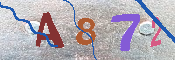## 送给爱雪的孩子“柯西雪花”–还是《写给下一代的电脑工程师》

331，东北(辽宁地区)下了一场雪，小保尔在外公家度假刚好赶上这场雪。小保尔的外公打电话回来给我们说：小保尔看到下雪了，高兴得跑出去玩雪；他说雪花很大，“象假的一样大”。

http://dongren.cn/NextProgramers/Koch/koch2snow2.gif“柯西雪花”漂亮吗？

BASICBeginner’s All-purpose Symbolic Instruction Code，初学者指令符号编码），意思就是初学者”使用的计算机语言。http://dongren.cn/NextProgramers/Koch/ColorSnows1.jpg

 BASICBeginner’s All-purpose Symbolic Instruction Code，又译培基），意思就是初学者的全方位符式指令代码，是一种设计给初学者使用的程序设计语言BASIC是一种解译式的程式语言，在完成编写后不须经由编译连结等手续即可执行，但如果需要单独执行时仍然需要将其建立成执行档。还要留些资料你自己阅读哦。http://zh.wikipedia.org/w/index.php?title=BASIC&variant=zh-hans

### “送给爱雪的孩子“柯西雪花”–还是《写给下一代的电脑工程师》”有一篇评论

1. baoer 评论道：

”柯西雪花“Koch’snow的主要迭代算法写一下,希望对学编程的有点个启发吧！代码通常占篇幅，真有兴趣咱们用SVN或邮箱来交流代码吧！

If (bX - aX) * (bX - aX) + (bY - aY) * (bY - aY) < 10 Then
‘Me.Line (aX, aY) - (bX, bY)’这一行是Vb6及以前版本的画线语句
g.DrawLine(mpen, aX, aY, bX, bY) ‘这一行是Vb.net的画线语句
Else
cX = aX + (bX - aX) / 3
cY = aY + (bY - aY) / 3
eX = bX - (bX - aX) / 3
eY = bY - (bY - aY) / 3
Call Fractal(aX, aY, cX, cY)
Call Fractal(eX, eY, bX, bY)
l = System.Math.Sqrt((eX - cX) * (eX - cX) + (eY - cY) * (eY - cY))
alpha = System.Math.Atan((eY - cY) / (eX - cX))
If (alpha >= 0 And (eX - cX) < 0) Or (alpha <= 0 And (eX - cX) < 0) Then
alpha = alpha + PI
End If
dY = cY + System.Math.Sin(alpha + PI / 3) * l
dX = cX + System.Math.Cos(alpha + PI / 3) * l
Call Fractal(cX, cY, dX, dY)
Call Fractal(dX, dY, eX, eY)
End If

做上图时优化了一下程序，PI用355 / 113“密率”代替了(为了迭代快），考虑程序可读性还是帖原来的。

Email: i90-#hotmail.com
#号替换掉。*